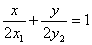Monday 08th August 2022CBSE Guess > Papers > Important Questions > Class XI > 2010 > Maths > Maths By Mr. Anil Kumar Tondak CBSE CLASS XI
Straight Lines Q.1. Find the value of K such that the line joining the points (2, K) and (-1, 3) is parallel to the line joining (0, 1) and (-3, 1). Q.2. Show that points (a, b + c), (b, c + a), (c, a + b) are collinear. Q.3. Show that points (at12 , 2at1) , (at22 , 2at2) and (a , 0 ) are collinear if t1t2 = - 1 Q.4. The slope of a line is double of the slope of another line. If tangent of the angle between them is 1/3, find the slope of the lines. Q.5. Find the equation of the straight line passing through (4,-2) and making an angle of 600 with the negative direction of Y axis. Q.6. A line perpendicular to the line segment joining the points (1,0) and (2,3) divides it in the ratio 1:n. Find the equation of the line. Q.7. The slope of a line is double of the slope of another line. If tangent of the angle between them is 1/3, find the slope of the lines. Q.8. Three points P (h, k), Q ( x1, y1) and R (x2, y2) lie on a line. Show that (h x1) ( y2 y1) = (k y1) (x2 x1). Q.9. Find the equation of the line that has y intercept 4 and is parallel to the line 2x 3y =7 Q.10. Find the equation of the line that has x intercept - 3 and is perpendicular to line 3x+5y =4. Q.11. Prove that the lines 7x 2y + 5 = 0 and 14x 4y 8 = 0 are parallel to each other. Q.12. Prove that the lines 3x 2y + 5 = 0 and 4x + 6y 23 = 0 are perpendicular. Q.13. The slope of a line is double of the slope of another line. If tangent of the angle between them is 1/3, find the slope of the lines. Q.14. A line perpendicular to the line segment joining the points (1,0) and (2,3) divides it in the ratio 1:n. Find the equation of the line. Q.15. Find the coordinates of the orthocentre of the triangle formed by the lines x+y-6=0 and 3y = 5x + 2. Q.16. Find the equation of a line that cuts off equal intercepts on the coordinate axes and passes through the point (2, 3). Q.17. Find the equation of a line which passes through the point (3, - 2) and is inclined at 600 to the line √3x + y = 1. Q.18. Find the equation of a line which passes through the point (x1 , y1) and perpendicular to the line xy1 + x1y = a2 Q.19. Find equation of the line passing through the point (2,2) and cutting off intercepts on the axes whose sum is 9. Q.20. Reduce the equation Ö3 x + y 8 = 0 into normal form. Find the value of w and p. Q.21. If p and q are the lengths of perpendiculars from the origin to the lines x cosq y sinq = k cos 2q and x sec q + y cosec q = k, respectively, prove that p2 + 4 q2 = k2. Q.22. A line such that its segment between the axis is bisected at the point (x1, y1). prove that the equation of the line isQ.23. In the triangle ABC with vertices A (2, 3), B (4, 1) and C (1, 2), find the equation and length of altitude from the vertex A. Q.24. Find equation of the line which is equidistant from parallel lines 9x + 6y -7 =0 and 3x + 2y +6=0 Paper By Mr. Anil Kumar Tondak Email Id : [email protected] Ph No.: 9811363962